# ShuffleNet-V2 Trained onImageNet Competition Data

Identify the main object in an image

Released in 2018, this model features pointwise group convolutions and bottleneck-like structures. A "channel shuffle" operation is introduced to enable information flow between different groups of channels and improve accuracy.

Number of layers: 243 | Parameter count: 2,294,784 | Trained size: 10 MB |

## Performance

• This model achieves a top-1 accuracy of 69.4% on the original ImageNet validation set.

## Examples

### Resource retrieval

Get the pre-trained net:

 In:=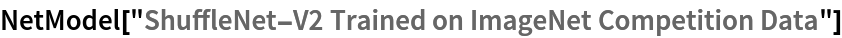Out=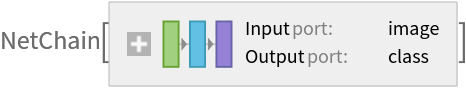### Basic usage

Classify an image:

 In:=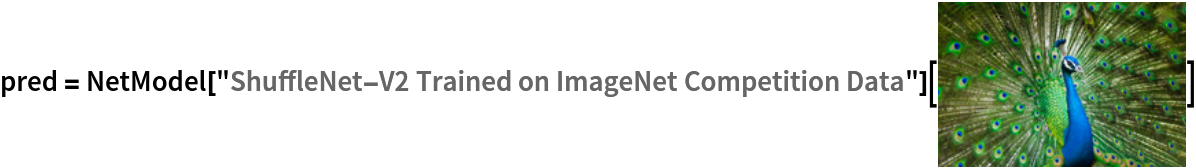Out=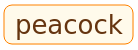The prediction is an Entity object, which can be queried:

 In:=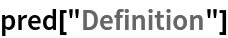Out=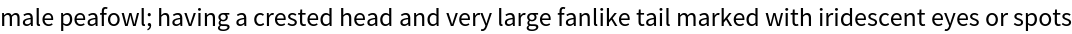Get a list of available properties of the predicted Entity:

 In:=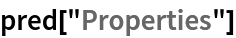Out=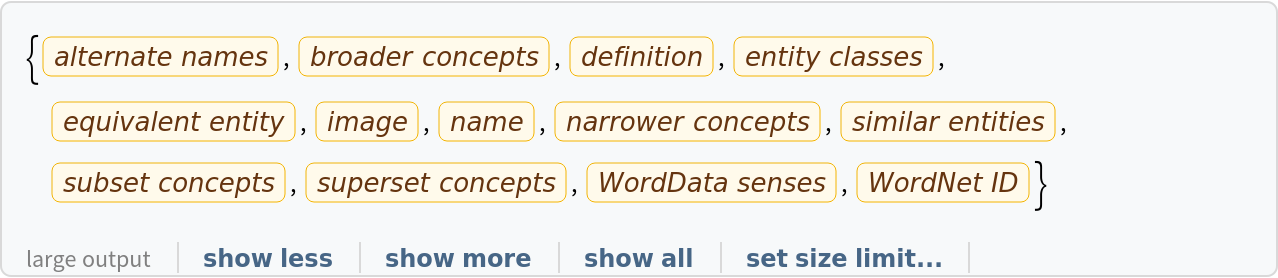Obtain the probabilities of the 10 most likely entities predicted by the net:

 In:=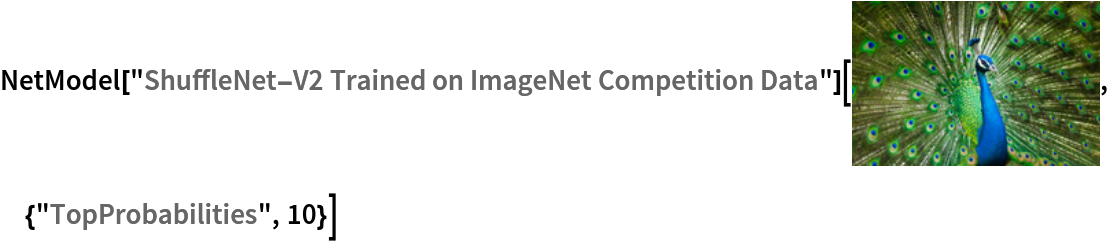Out=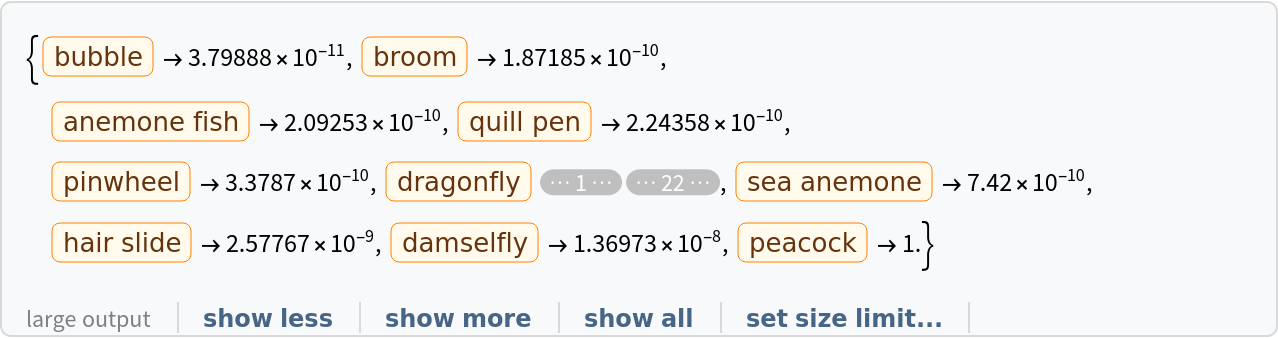An object outside the list of the ImageNet classes will be misidentified:

 In:=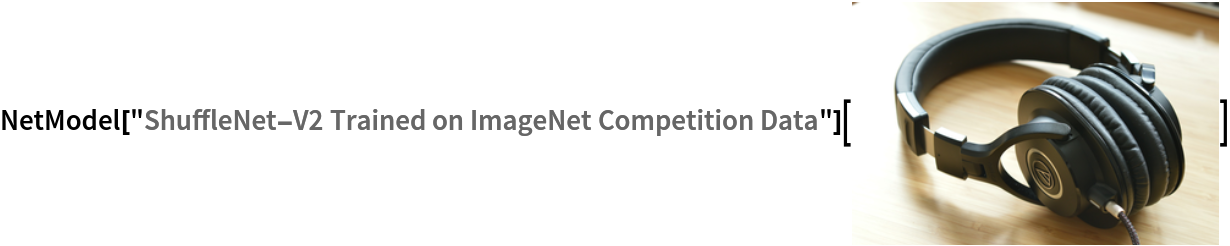Out=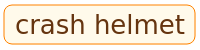Obtain the list of names of all available classes:

 In:=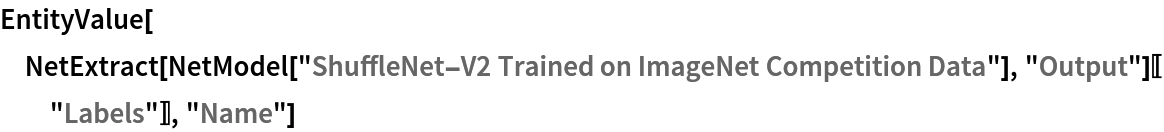Out=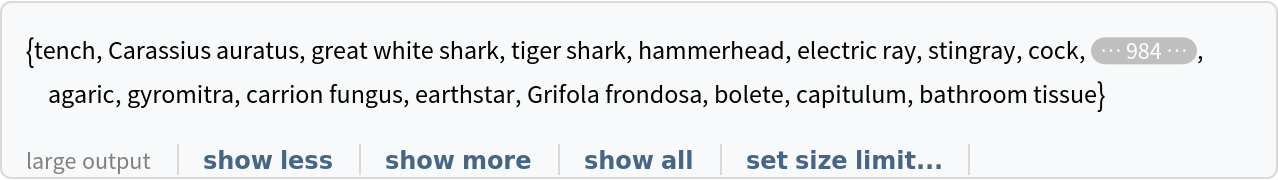### Feature extraction

Remove the last layers of the trained net so that the net produces a vector representation of an image:

 In:=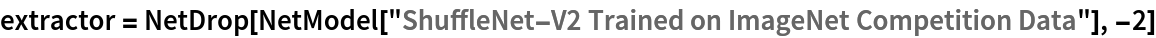Out=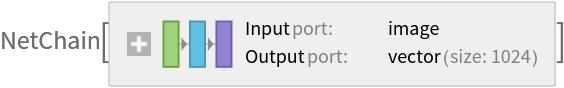Get a set of images:

 In:=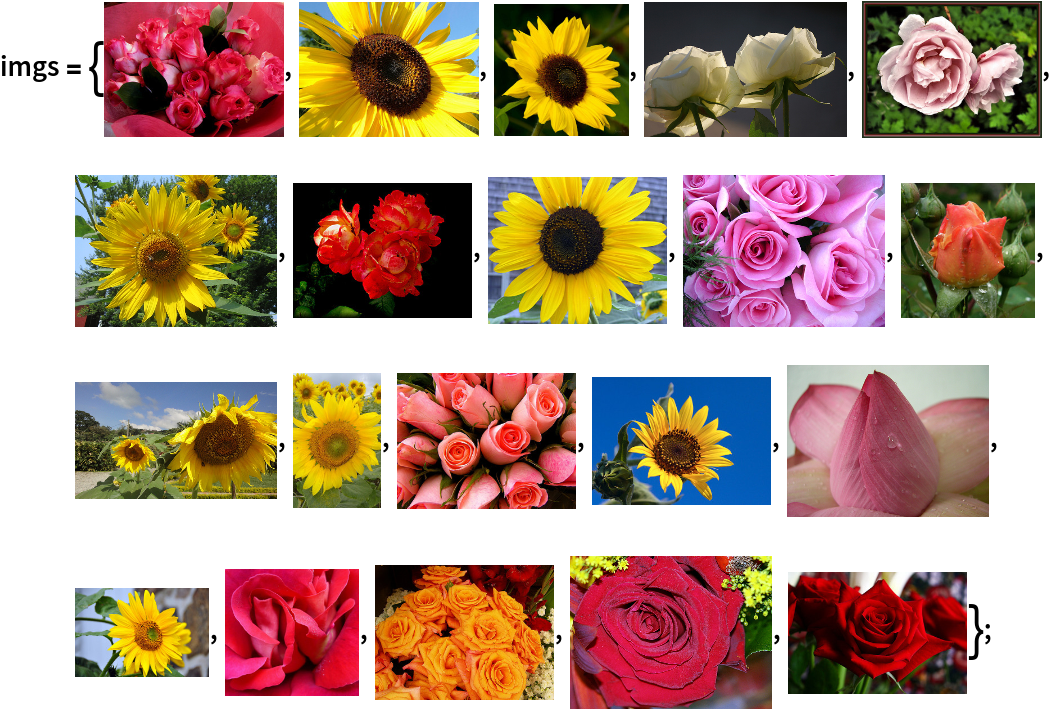Visualize the features of a set of images:

 In:=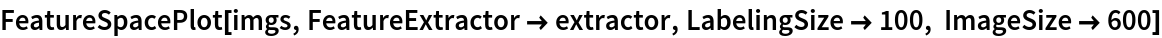Out=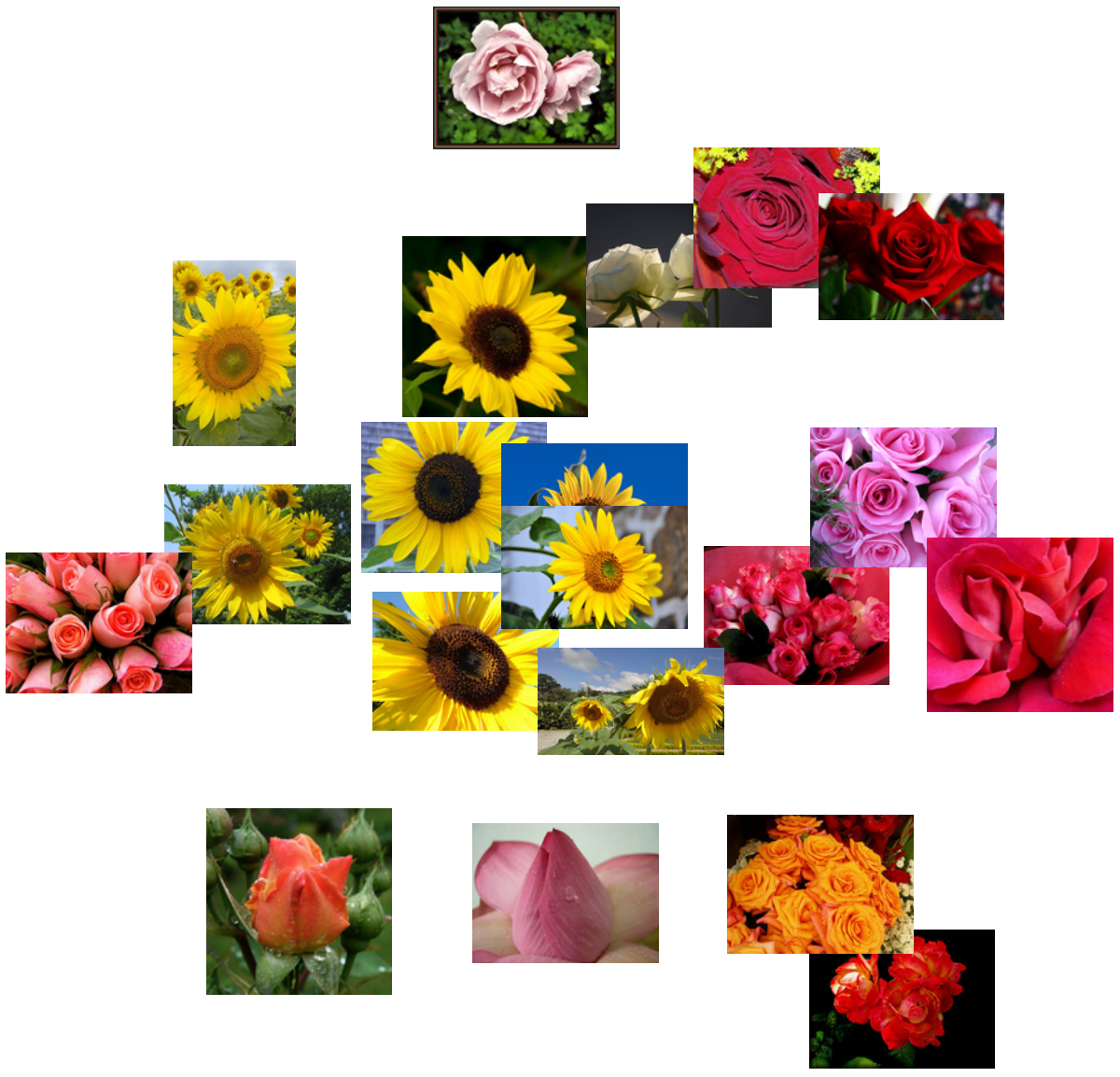### Visualize convolutional weights

Extract the weights of the first convolutional layer in the trained net:

 In:=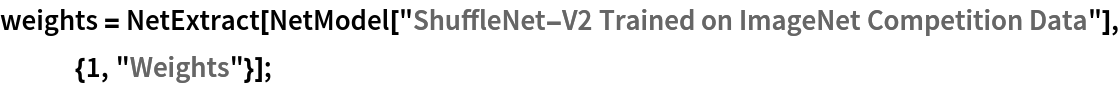Show the dimensions of the weights:

 In:=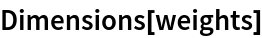Out=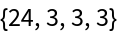Visualize the weights as a list of 24 images of size 3⨯3:

 In:=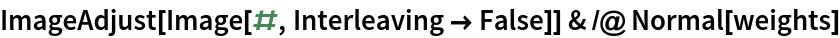Out=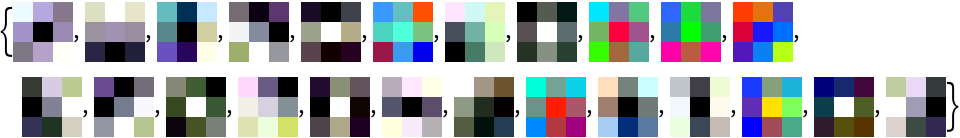### Transfer learning

Use the pre-trained model to build a classifier for telling apart images of sunflowers and roses. Create a test set and a training set:

 In:=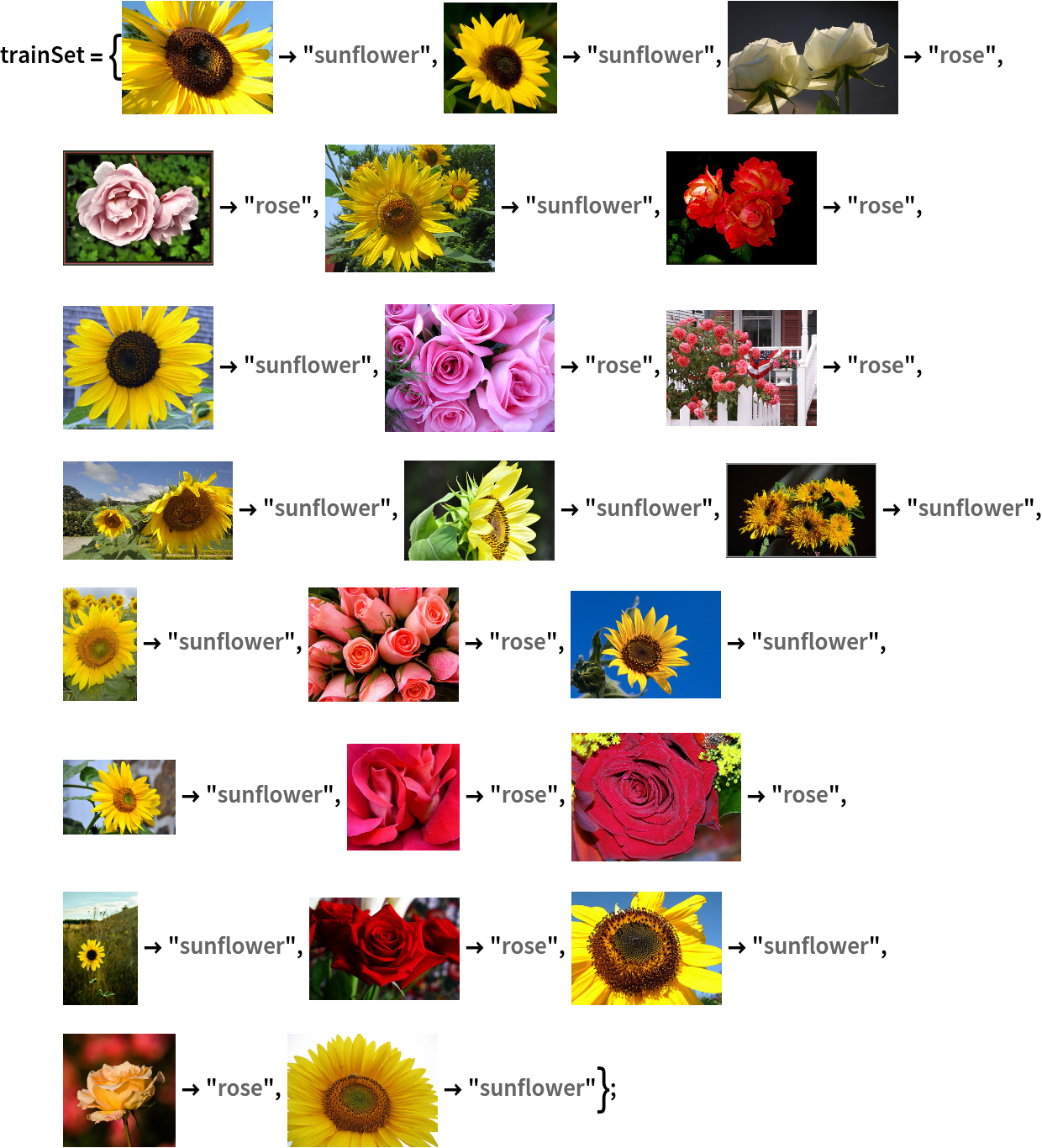In:=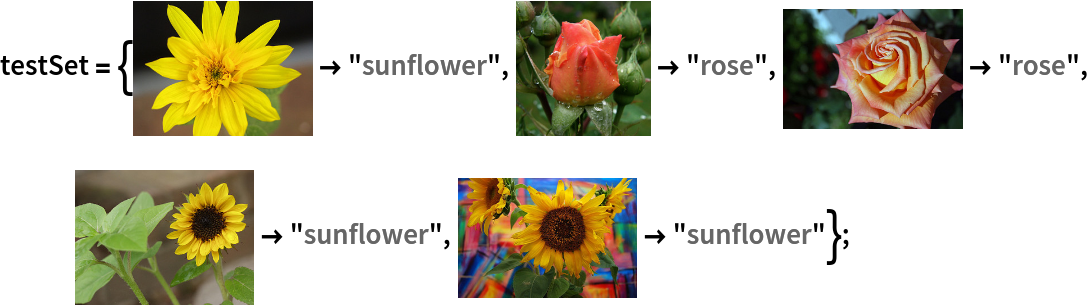Remove the last layers from the pre-trained net:

 In:=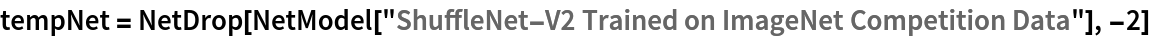Out=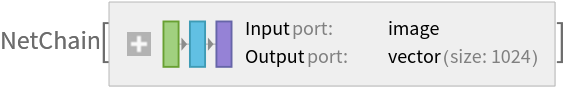Create a new net composed of the pre-trained net followed by a linear layer and a softmax layer:

 In:=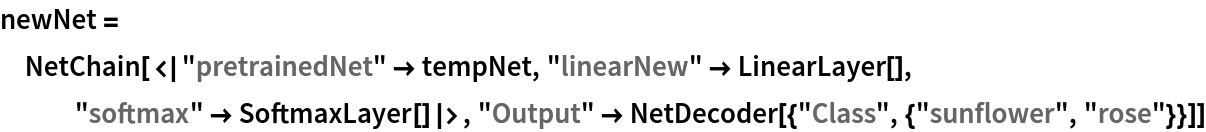Out=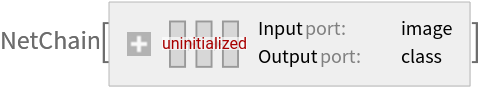Train on the dataset, freezing all the weights except for those in the "linearNew" layer (use TargetDevice -> "GPU" for training on a GPU):

 In:=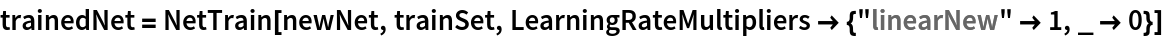Out=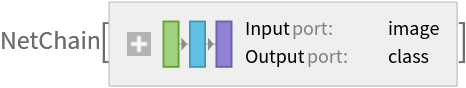Accuracy obtained on the test set:

 In:=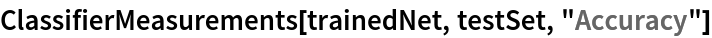Out=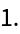### Net information

Inspect the number of parameters of all arrays in the net:

 In:=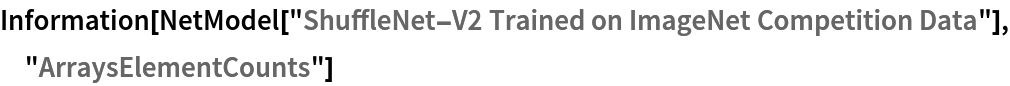Out=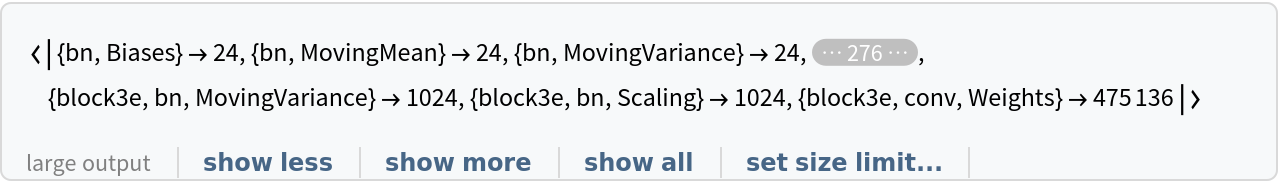Obtain the total number of parameters:

 In:=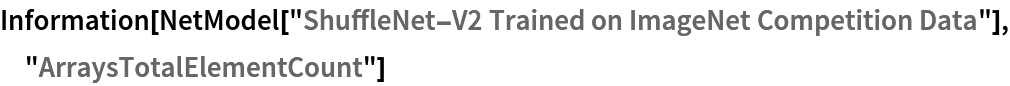Out=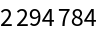Obtain the layer type counts:

 In:=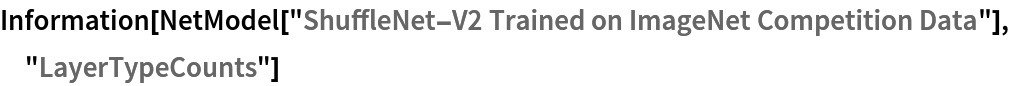Out=Display the summary graphic:

 In:=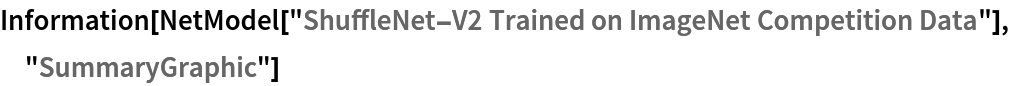Out=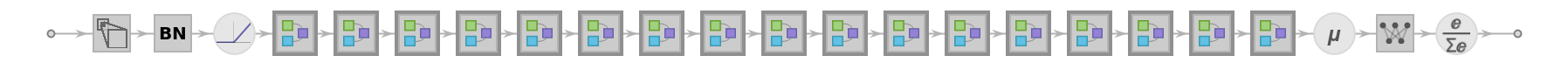### Export to ONNX

Export the net to the ONNX format:

 In:=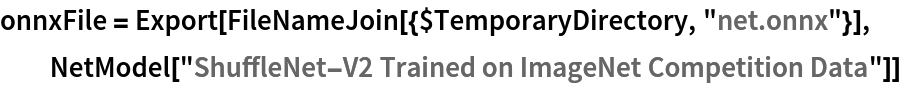Out=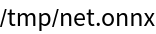Get the size of the ONNX file:

 In:=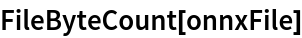Out=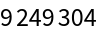The size is similar to the byte count of the resource object:

 In:=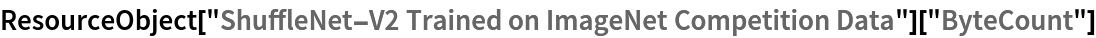Out=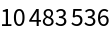Check some metadata of the ONNX model:

 In:=Out=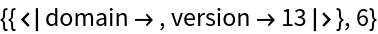Import the model back into the Wolfram Language. However, the NetEncoder and NetDecoder will be absent because they are not supported by ONNX:

 In:=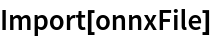Out=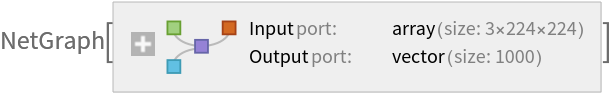## Requirements

Wolfram Language 12.3 (May 2021) or above

## Reference

• N. Ma, X. Zhang, H.-T. Zheng, J. Sun, "ShuffleNet V2: Practical Guidelines for Efficient
CNN Architecture Design," arXiv:1807.11164v1 (2018)
• Rights: BSD 3-Clause